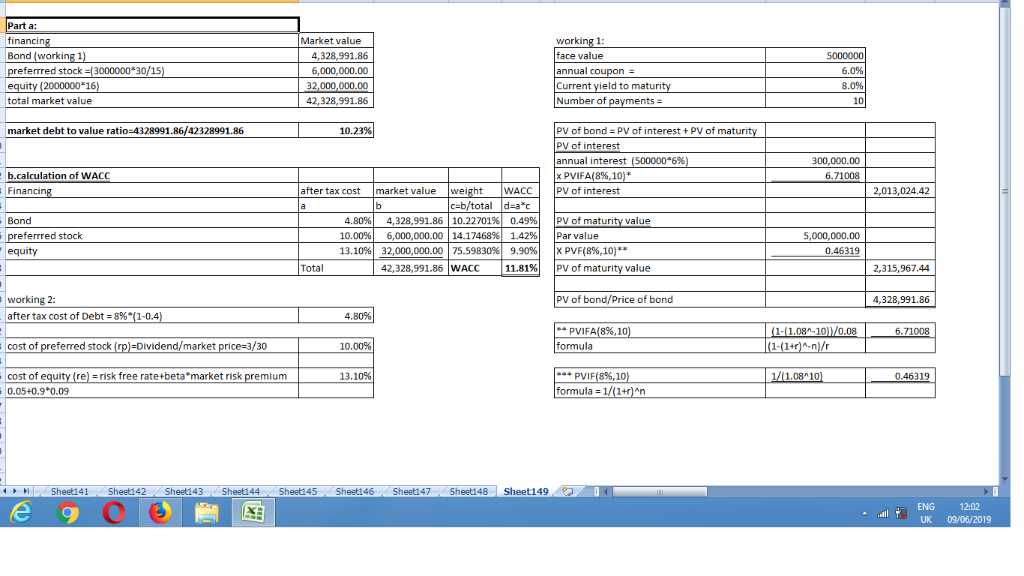In: Finance

# Examine the following book-value balance sheet for University Products Inc. The preferred stock currently sells for...

Examine the following book-value balance sheet for University Products Inc. The preferred stock currently sells for $30 per share and pays a dividend of$3 a share. The common stock sells for $16 per share and has a beta of 0.9. There are 2 million common shares outstanding. The market risk premium is 9%, the risk-free rate is 5%, and the firm’s tax rate is 40%.  BOOK-VALUE BALANCE SHEET (Figures in$ millions) Assets Liabilities and Net Worth Cash and short-term securities $2.0 Bonds, coupon = 6%, paid annually (maturity = 10 years, current yield to maturity = 8%)$ 5.0 Accounts receivable 3.0 Preferred stock (par value $15 per share) 3.0 Inventories 7.0 Common stock (par value$0.20) 0.4 Plant and equipment 21.0 Additional paid-in stockholders’ equity 13.6 Retained earnings 11.0 Total $33.0 Total$ 33.0

a. What is the market debt-to-value ratio of the firm? (Do not round intermediate calculations. Enter your answer as a percent rounded to 2 decimal places.)

b. What is University’s WACC? (Do not round intermediate calculations. Enter your answer as a percent rounded to 2 decimal places.)

## Solutions

##### Expert Solution## Related Solutions

##### Examine the following book-value balance sheet for University Products Inc. The preferred stock currently sells for...
Examine the following book-value balance sheet for University Products Inc. The preferred stock currently sells for $15 per share and pays a dividend of$3 a share. The common stock sells for $20 per share and has a beta of 0.8. There are 2 million common shares outstanding. The market risk premium is 8%, the risk-free rate is 4%, and the firm’s tax rate is 21%. BOOK-VALUE BALANCE SHEET (Figures in$ millions) Assets Liabilities and Net Worth Cash and...
##### Examine the following book-value balance sheet for University Products Inc. The preferred stock currently sells for...
Examine the following book-value balance sheet for University Products Inc. The preferred stock currently sells for $15 per share and pays a dividend of$3 a share. The common stock sells for $20 per share and has a beta of 0.7. There are 2 million common shares outstanding. The market risk premium is 12%, the risk-free rate is 8%, and the firm’s tax rate is 21%. BOOK-VALUE BALANCE SHEET (Figures in$ millions) Assets Liabilities and Net Worth Cash and...
##### Examine the following book-value balance sheet for University Products Inc. The preferred stock currently sells for...
Examine the following book-value balance sheet for University Products Inc. The preferred stock currently sells for $30 per share and pays a dividend of$3 a share. The common stock sells for $20 per share and has a beta of 0.7. There are 2 million common shares outstanding. The market risk premium is 12%, the risk-free rate is 8%, and the firm’s tax rate is 40%. BOOK-VALUE BALANCE SHEET (Figures in$ millions) Assets Liabilities and Net Worth Cash and...
##### Examine the following book-value balance sheet for University Products Inc. The preferred stock currently sells for...
Examine the following book-value balance sheet for University Products Inc. The preferred stock currently sells for $30 per share and pays a dividend of$3 a share. The common stock sells for $20 per share and has a beta of 0.8. There are 1 million common shares outstanding. The market risk premium is 12%, the risk-free rate is 8%, and the firm’s tax rate is 40%. BOOK-VALUE BALANCE SHEET (Figures in$ millions) Assets Liabilities and Net Worth Cash and...